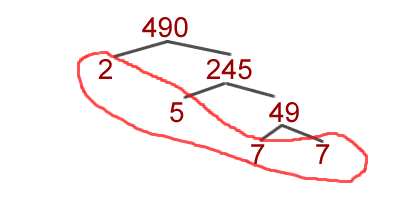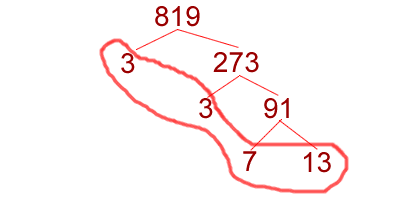# The largest factor shared by two numbers

### Definition

The greatest common factor is, like it sounds, the largest number that factors evenly into two or more larger numbers. For instance, the greatest common factor (GCF) of 15 and 25 is 5, because 5 is the largest number that goes into 15 and 25 evenly.

To find the GCF of small numbers, like 12 or 16, it might be easier to just list all the factors and find the largest common factor, but for big numbers like 490 and 819, you need a faster method. The first step is to complete a prime factorization of each number. Find any number (2 will work) that divides 490 evenly, and you will get 245. Keep dividing and keeping track of the numbers in a format like this:Therefore, 7 * 7 * 5 * 2 = 490. Those four numbers circled in red are all prime and cannot be factored anymore, so you must be done. That is the prime factorization of 490. Now you can do the same with your other number, 819.The prime factors of 819 are 3,3,7, and 13. Now we should compare the prime factors of both numbers:

$$819 = 3 * 3 * 7 * 13$$ $$490 = 2 * 5 * 7 * 7$$

What we want to do is take everything that is shared by both numbers. Only one factor is common, and that is 7, so we know that 7 is the GCF of 490 and 819. Let's try another pair of numbers, 1012 and 10580. Prime factorization of the numbers reveals this situation:

$$1012 = 2 * 2 * 11 * 23$$ $$10580 = 2 * 2 * 5 * 23 * 23$$

Each number has two 2's and one 23 in common, so we will use those numbers. We cannot use the other 23 because 1012 only has one, and we cannot use 11 because 10580 doesn't have any. Write down the numbers in common and multiply them to get the GCF:

$$2 * 2 * 23 = 92$$

That's all there is to finding the Greatest Common Factor (GCF) of a number. If you need more help, please visit another lesson on greatest common factors, browse our site, or use a search engine like Google to find more information.